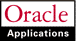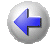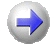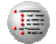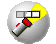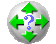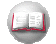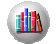Previous Next Contents Index Navigation Glossary Library

## Oracle FastFormula Overview

Formulas are generic expressions of calculations or comparisons you want to repeat with different input values. They can take input from the window, database, or a process, such as a payroll run. They can return values or messages.

You can use formulas to:

• calculate element pay values and run results during payroll processing
• check that element entry values are valid for an assignment
• check that entries made to a user table are valid
• specify the criteria for including an assignment in an assignment set
• select the database information you want to display in a QuickPaint report, and perform calculations for the report
• specify the rules for skipping an element during payroll processing
• prepare records in the format you require for the magnetic tape writer. For most countries, the Oracle localization team has written the required formulas.
When you write a formula, you specify for which of these purposes you will use it.

Formulas for QuickPaint reports and assignment sets can be generated from criteria you enter in windows. You can edit these generated formulas to add more functionality.

## Writing a Simple Payroll Calculation

There are strict rules as to the words and punctuation you must use in a formula so that Oracle FastFormula can correctly perform the calculations and validation you require.

To start with a simple example, suppose you wanted to calculate the pay value for the element Wage by multiplying the number of hours an employee works each week by his hourly rate. You could write this formula:

`	wage = hours_worked * hourly_rate`
`	RETURN wage`

The first line is an Assignment statement that simply assigns a value to the element Wage. The second line is a Return statement that passes back the Wage value to the payroll run.

In this example, the Wage value is calculated, but it could be a constant value, such as: wage = 200. To calculate the Wage value, Oracle FastFormula needs to get values for the variables hours_worked and hourly_rate. They are called variables because they can contain different values depending, in this example, on which assignment the payroll run is processing.

### Data Types

Both variables and constants can be one of three data types:

• numeric
• text
• date
The variables in the Wage example are numeric.

## Types of Input

We have said that Oracle FastFormula needs to get values for the variables hours_worked and hourly_rate. There are three ways it can get values:

• it can receive them as input when the formula is called
• it can find the values in the database from database items
• it can use global values, which you enter in the Globals window
To use a database item or global value in your formula, you simply refer to it by name. You can browse through lists of database items in the Formulas window. To use a value passed to the formula at run time, you must write an Inputs statement.

### Using Inputs Statement

In our Wage example, suppose that hours_worked is an input value to the element Wage. To pass the element input values to the formula during a payroll run, you define an Inputs statement, as follows:

`	INPUTS ARE hours_worked`
`	wage = hours_worked * hourly_rate`
`	RETURN wage`

The name you use in the Inputs statement must be the same as the name of the element input value, and multiple words must be joined by underscores. In this example, the input value hours_worked is numeric. If the input value is not numeric, you must tell Oracle FastFormula whether it is text or date. For example:

`	INPUTS ARE start_date (date)`

### Using Database Items

Suppose that hourly_rate is a standard rate taken from the Grade Rates table. This is an example of a database item. A database item has a label, or special piece of code, telling Oracle FastFormula the path to take to access the data. Oracle HRMS produces most of the database items you need without you taking any special action. These items include both information unique to your enterprise, which you hold in flexfield segments, as well as standard information such as assignment numbers and grades.

In the Formulas window, you pick database items from a list. You will see that the name of the database item for a grade rate called hourly_rate is actually grade_hourly_rate_value. This is the name you must use in your formula.

By this naming convention, Oracle FastFormula knows that hourly_rate is a database item from the Grade Rate table. But how does it know which hourly_rate to use from this table? It works this out from the context the payroll run provides for each element entry. The context identifies:

• the element and element link
• the payroll and payroll run
• the employee and employee assignment.
Attention: You should use an Inputs statement in preference to database items where possible because this is more efficient.

See: Writing Efficient Payroll Calculation Formulas.

### Using Global Values

Use global values to store information that does not change often, but you refer to frequently, such as Company Name, or company-wide percentages used to calculate certain types of bonus. You define the global value and change its value using the Globals window.

### Using Local Variables

Local variables exist in one formula only. You can change the value of a local variable by assigning it a value in an Assignment statement. In the Wage example, the variable wage itself is a local variable. It receives a value within the formula by the Assignment statement:

`	wage = hours_worked * hourly_rate`

Note: You cannot change the value of input values, database items, or global values within a formula.

## Writing More Complex Calculations

The Assignment statement in the wages example above uses a simple multiplication to calculate the value of the wages element. You can also use addition, subtraction, division, and a number of functions. For example:

`	bonus = GREATEST(days_at_work,163) + bonus_rate`

Here the function GREATEST tells Oracle FastFormula to use the value of the variable days_at_work, if it is greater than 163, and otherwise to use the constant 163.

The data type of variables and constants determines how operators and functions act on the values. For example, the addition operator (+) can be used with numeric or text data, while division can be used with numeric data only.

There are special functions that convert variables from:

• numbers to text (TO_TEXT)
• dates to text (TO_TEXT)
• text to date (TO_DATE)
• text to number (TO_NUM)
See: Functions

### Nested Expressions

The Assignment statement can use as many arithmetic operators and functions as you require. Each function or calculation is one expression, and you can nest expressions to create more complex calculations. You must use brackets to make clear to Oracle FastFormula the order in which the calculations are performed. For example:

`	ANNUAL_BONUS = trunc(((((salary_amount/100)*`
`	bonus_percentage)/183)*(days_between(end_period_date,`
`	start_date) + 1)), 2)`

Oracle FastFormula begins calculating inside the brackets and from left to right, in the following steps:

1. salary_amount/100
2. 1. * bonus_percentage
3. 2. / 183
4. days_between (end_period_date, start_date)
5. 4. + 1
6. 3. * 5.
7. TRUNC(6.,2)
Notice that TRUNC is another function. It rounds a numeric value down to the number of decimal places specified after the comma (two in this case).

## Incorporating Conditions

In our simple Wage element example, only one value is returned, and it is calculated in the same way for every assignment. However you may need to perform different calculations depending on the particular group of employee assignments, or the time of the year, or some other factors. You can do this by incorporating conditions in your formula.

### Using Simple Conditions

For example:

`	IF age < 20 THEN`
`	  training_allowance = 30`
`	ELSE`
`	  training_allowance = 0`

The formula checks whether the condition (age < 20) is true or false. If it is true, the formula processes the statement that follows the word THEN. If the condition is not true, the formula ignores this statement and processes any statement that follows the word ELSE. The ELSE clause is optional.

### Making Conditions More Complex

In the example above, the condition compares a variable (age) to a constant (20). The condition can be more complex, comparing expressions that contain functions and arithmetic operators. For example:

`	IF (DAYS_BETWEEN(end_period_date, start_date)+1) >= threshold`

DAYS_BETWEEN is another function.

We have seen two comparators: less than (<) and greater than or equal to (>=). A full list of the comparators you can use appears in the Reference section.

### WAS DEFAULTED

There is a special type of condition called WAS DEFAULTED. Use this to test whether a default value has been placed in an input value or database item. Default values are placed using the Default statement. For example:

`	DEFAULT FOR hourly_rate IS 3.00`
`	X = hours_worked * hourly_rate`
`	IF hourly_rate WAS DEFAULTED`
`	  THEN`
`	    MESG = 'Warning: hourly rate defaulted'`

In this example, if the database item hourly_rate is empty (NULL), the formula uses the default value of 3.00 and issues a warning message.

### Combining Conditions

You can combine conditions using the logical operators AND, OR, NOT.

• Use AND if you want an action to occur when more than one condition is true. For example:
`	IF  (days_between(end_period_date, start_date) + 1) >= 183`
`	AND  employee_status = 'FULL TIME'`
`	THEN  . . .`

• Use OR if you want an action to occur when any one of two or more conditions is true. For example:
`	IF  stock_level < 10000`
`	OR  order_size >= 1500`
`	THEN  . . .`

• Use NOT if you want an action to occur when a condition is not true. For example:
`	IF NOT (months_between(purchase_date, system_date) => 60`
`	THEN  . . .`

As with Assignment statements, you may need to use brackets to tell Oracle FastFormula in which order to test conditions. By default, NOT has the highest precedence, followed by AND then OR. So the following condition:

`	IF   X = 6 AND NOT Y = 7 OR P >= 6`

is interpreted as:

`	IF   (X = 6 AND (NOT Y = 7)) OR P >= 6`

How you use brackets can change dramatically the meaning of a formula.

Suggestion: Use brackets whenever you create multiple conditions, so that the meaning of the formula is clear to other readers.

### Incorporating Multiple Actions based on Conditions

We have seen how to make conditions more complex. You can also make the actions performed as complex as you like. In our simple example above, the result of the condition was the assignment of a value to the variable training_allowance. As well as assigning values, you can perform calculations and return values.

For example, suppose you must check whether there are sufficient funds in a bank account before processing a withdrawal:

`	INPUTS ARE acct_balance,`
`	           acct (text),`
`	           debit_amt`
`	IF   acct_balance >= debit_amt`
`	  THEN`
`	  (`
`	     new_balance = acct_balance - debit_amt`
`	     RETURN new_balance`
`	  )`
`	  ELSE `
`	  (`
`	     message = 'Account No. ' + acct + ' - Insufficient Funds'`
`	     message2 = 'Account Balance is ' + TO_TEXT(acct_balance)`
`	     RETURN message, message2`
`	  )`

Notice that you can return two variables in the same Return statement.

Attention: The brackets following THEN and ELSE are essential when you have multiple actions based on a condition. Without them, Oracle FastFormula processes the first statement conditionally and the other statements unconditionally.

It is good practice to include comments in your formulas to explain to other people what the formula does.

So, for example, you can name your formula:

`	/*  Formula:  Attendance Bonus  */`

and write an explanation of your formula:

```	/*  Use this formula to calculate the annual bonus for
clerical staff.  Employees receive either a percentage of
their salary (if they have been present for 183 or more
days in the last six months), or a pro rata bonus (if they
have been in attendance for less than 183 days in the last
six months).  */```

Oracle FastFormula ignores everything between the comment delimiters: /* and */. You can place comments anywhere in a formula without affecting the formula's performance.

Warning: Do not put a comment within a comment. This causes Oracle FastFormula to return a syntax error.

You can use a comment to explain what part of your formula does. So, for example, you might want a comment explaining who decides the bonus percentage:

`	INPUTS ARE  salary_amount,`
`	            start_date (date),`
`	            end_period_date (date),`
`	            bonus_percentage /*  decided at board level */`

You can also use comments to `comment out' parts of the formula you do not currently want to use. So, for example, you can include a fifth input of employee_status, ensuring that employees with a status of full time are awarded a bonus. However, as you do not yet have a range of statuses, you do not currently need the fifth input.

`	INPUTS ARE  salary_amount,`
`	            start_date (date),`
`	            end_period_date (date),`
`	            bonus_percentage /*  decided at board level  */`
`	        /*  employee_status (text)  */`

## Formula Structure

A valid formula has a well defined structure. It is made up of comments and statements. Oracle FastFormula ignores comments and carries out the instructions in the statements.

We have already looked at four types of statement:

• Assignment statement
• Inputs statement
• Return statement
• If statement
There are two other types of statement you can use:

• Alias statement
• Default statement

### Alias Statement

Database items are named by the system when it creates them, and sometimes these names are too long to conveniently use in a formula. You cannot shorten the name of a database item (or a global value) itself, but you can set up an alternative shorter name to use within the formula. For example:

`	ALIAS  as_overtime_qualifying_length_of_service AS ot_qls`

In the rest of the formula, you can use the alias (in this example, ot_qls) as if it were the actual variable.

Attention: Using an Alias is more efficient than assigning the database item to a local variable with a short name.

### Default Statement

Use the Default statement to set a default value for an input value or a database item. The formula uses the default value if the database item is empty or no input value is provided when you run the formula. For example:

`	DEFAULT FOR hourly_rate IS 3.00`
`	X = hours_worked * hourly_rate`
`	IF hourly_rate WAS DEFAULTED`
`	  THEN`
`	    MESG = 'Warning: hourly rate defaulted'`

This example sets a default of 3.00 for the database item hourly_rate. If hourly_rate is empty (NULL) in the database, the formula uses the default value of 3.00. The formula uses the 'WAS DEFAULTED' test to detect when a default value is used, in which case it issues a warning message.

Attention: You must use the Default statement for database items that can be empty. The Database Items window includes a check box labelled Default Required. This check box is checked for database items that can be empty. The Database Items window appears when you choose the Show Items button on the Formulas window.

### Order of Statements

Statements in your formula must appear in the following order:

1) ALIAS statements (if any)

2) DEFAULT FOR statements (if any)

3) INPUT statement (if any)

4) Other statements